## Concept – Canopy

The Sierpiński triangle, also called the Sierpiński gasket or Sierpiński sieve, is a fractal attractive fixed set with the overall shape of an equilateral triangle, subdivided recursively into smaller equilateral triangles. Originally constructed as a curve, this is one of the basic examples of self-similar sets.

The fractal canopy, a type of fractal tree, is one of the easiest-to-create types of fractals. Each canopy is created by splitting a line segment into two smaller segments at the end, and then splitting the two smaller segments as well, and so on, infinitely.Canopies are distinguished by the angle between concurrent adjacent segments and ratio between lengths of successive segments.

The design concept is basing on the solar radiation simulation of the Mies van der Rohe pavilion, by applying both Sierpiński triangle and fractal canopy, using recursion to generate a Branching Structure for shading.Concept  Image credits: Sierpinski pyramid, en.wikipedia, JJLudemann

## Pseudo Code

The main modeling process are three steps:

1. First, simulate the solar radiation condition of design area.
2. Then, branching the meshes based on condition.
3. Finally, execute the recursion function based on the branches.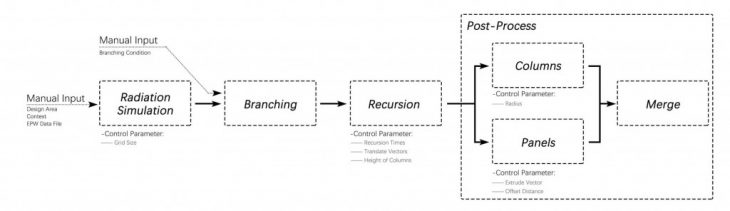Pseudo Code

## ScriptGrasshopper Script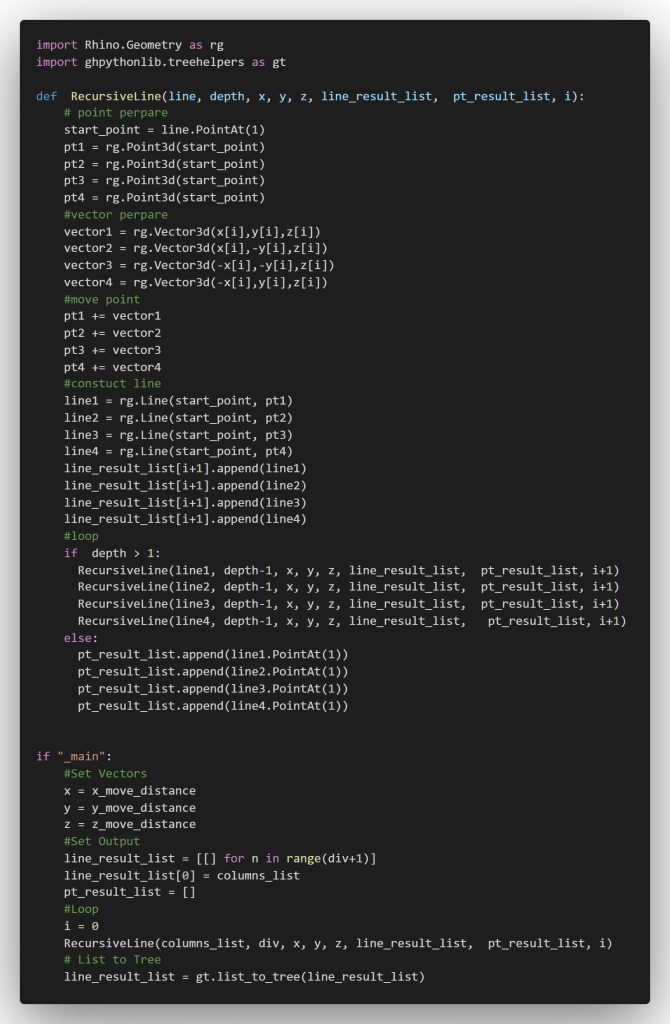Python Code

## Design Process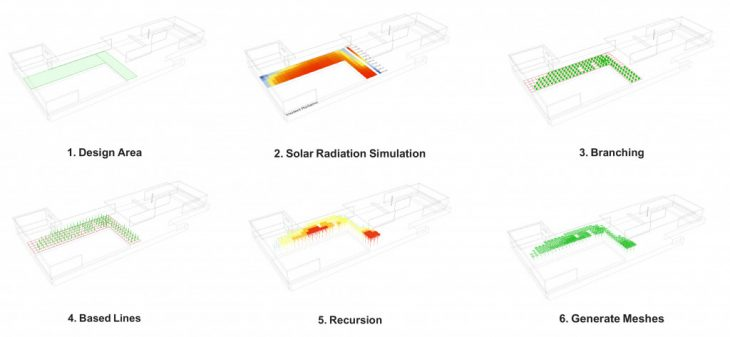Generation Process

1. Design Area: The input data is the design area, which will further used for solar radiation simulation.
2. Solar Radiation Simulation: Based on the EPW file, simulate the annual direct radiation on design area.
3. Branching: After that, by setting the branching condition and filtering the values, the meshes and corresponding radiation values are distributed into several branches.
4. Based Lines: Generate the based lines from each meshpoint.
5. Recursion: Execute and end the recursion based on the loop condition.
6. Generate Meshes: Finally, generate the columns and panels by input lines.

## Recursive Logic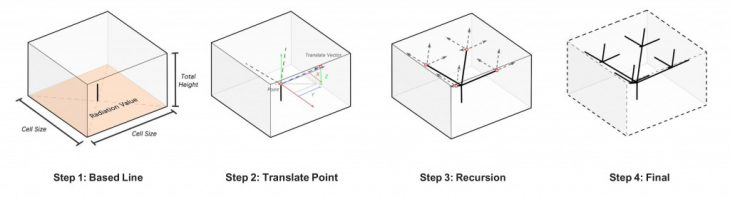Recursive Logic

1. At the beginning, generate a line at the center of the cell, this is the based geometry to recursion. In addition, a radiation value is linking to this line. The line will recurves based on the branching condition setting.
2. Based on the cell size, total height of the unit and the recursion times, calculate the corresponding coordinate value of the translate vector. Move the point with the vector and generate new lines for next loop.
3. Then repeat the function that defined in the previous step, until  match the ending condition.
4. Finally, recursion end, obtain the final result.

## CatalogueCatalogue

## Render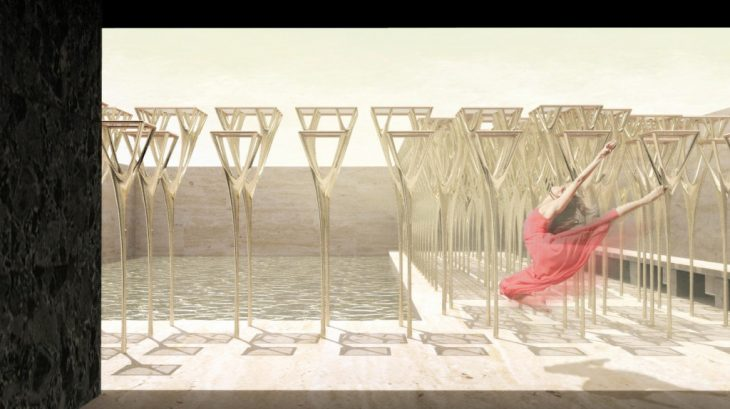Render

## Vedio

<p>

</p>

Algorithmic Emergence // The Canopy is a project of IaaC, Institute for Advanced Architecture of Catalonia developed at Master in Advanced Architecture in 2020/2021 by:
Student: Liang Mayuqi
Faculty: Rodrigo Aguirre
Assistant: Ashkan Foroughi Dehnavi